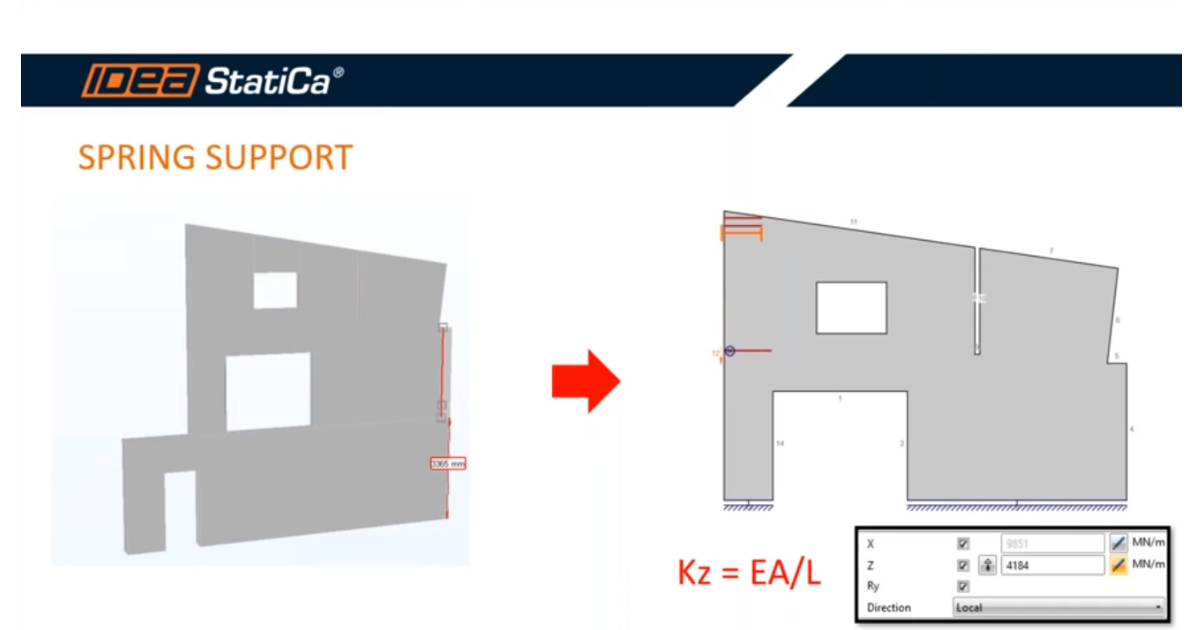### Choose language# How to input axial stiffness of the line support in the vertical direction Z

$$The complex behavior of the structure as redistribution of the stresses and also deformations are affected by the stiffness of the supports. We input the vertical stiffness in ,, Z,, direction to obtain a more precise model. The calculation of the axial stiffness can be expressed according to this equation. Equation… Kz = E*A/L$$